Question Pool Geometry and Algebra

# Geometry and Algebra - SAMAGRA Question Pool & Answers | Class 10 English Medium

Kerala Syllabus SAMAGRA SCERT SAMAGRA Question Pool for Class 10 English Medium Maths Geometry and AlgebraQn 1.

(a) Find Slope of the line passing through ( 3 ,5 ) , ( 4 , 7 ).

(b) What is the slope a line parallel to this line ?

a) Slope = 2

Get Free Study Materials + 1 Week Free Trial of BrainsPrep Class 10 English Medium Tuition

Qn 2.

Write the equation of the line passing through A ( 0 ,12) and B ( 16 , 0)

Get Free Study Materials + 1 Week Free Trial of BrainsPrep Class 10 English Medium Tuition

Qn 3.

The vertices of a triangle are ( -3 , 3 ) , ( 5 , 3 ) and ( 1 , 6 ) . Prove that it is an isosceles triangle

Lengths of sides are 8, 5, 5

Get Free Study Materials + 1 Week Free Trial of BrainsPrep Class 10 English Medium Tuition

Qn 4.

Consider the points A ( 1, 0 ) , B ( 7 , 0 ) C ( 4 , 4 )

a). Which of these points are on the x - axis ?

b). Prove that triangle ABC is isosceles.

a) A (1 , 0), B (7 ,

Get Free Study Materials + 1 Week Free Trial of BrainsPrep Class 10 English Medium Tuition

Qn 5.

Consider the points L ( 9, 2) and M (1 , -2)

a). What is the slope of the line LM ?

b). Find the coordinates of two more points on the line

c). Find the coordinates of the point where this line meets the x - axis

(a) slope =

Get Free Study Materials + 1 Week Free Trial of BrainsPrep Class 10 English Medium Tuition

Qn 6.

If A (2,3) and B (6,9) are two points on a line , then

(a) Find the coordinates of the mid point of the line AB

(b) Find the slope of AB

(c) Find the equation of the line having slope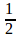and passing through the mid point of AB.

Get Free Study Materials + 1 Week Free Trial of BrainsPrep Class 10 English Medium Tuition

Qn 7.

P (5 , 2), Q (8 , 6) are two points on a line, then

a). What is the slope of PQ?

b). Write the equation of the line PQ

c). Find the co-ordinates of the point at which the line PQ cut the 'x' axis

Get Free Study Materials + 1 Week Free Trial of BrainsPrep Class 10 English Medium Tuition

Qn 8.

If the equation of a circle is x2+y2=4 then

(a)What is the radius of the circle ?

(b) If the x coordinate of a point on this  circle is zero, what is the y- coordinate of that point ?

(c) Write the coordinates of another point on the circle.

Get Free Study Materials + 1 Week Free Trial of BrainsPrep Class 10 English Medium Tuition

Qn 9.

A circle with centre at (1,3) passes through the point  (5,6).

(a) Find the radius of the circle?

(b) Write the equation of the circle.

Get Free Study Materials + 1 Week Free Trial of BrainsPrep Class 10 English Medium Tuition

Qn 10.

A (2,5) and B (a,-3) are joined to get the line AB as in the figure and P (4,b) is the mid point of AB.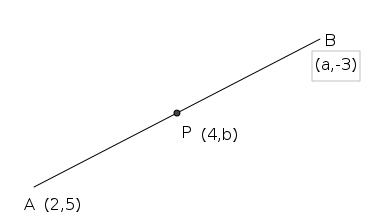a) Find the value of a .

b) Find the value of b .

a) a =

Get Free Study Materials + 1 Week Free Trial of BrainsPrep Class 10 English Medium Tuition

Qn 11.

The equation of a circle is x2+y2=9

(a) Find the radius of the circle ?

(b) Write the coordinates of the centre of the circle .

(c) Find the points of contact of the circle with X axis.

Get Free Study Materials + 1 Week Free Trial of BrainsPrep Class 10 English Medium Tuition

Qn 12.

A (2,3) B (6,7) are two points on a line .

(a) Find the slope of AB.

(b) If P is the mid point of AB , then find the coordinates of P.

(c) Write the equation of the line AB.

Get Free Study Materials + 1 Week Free Trial of BrainsPrep Class 10 English Medium Tuition

Qn 13.

In the figure PB : QB = 1 : 2. Find the coordinates of the point B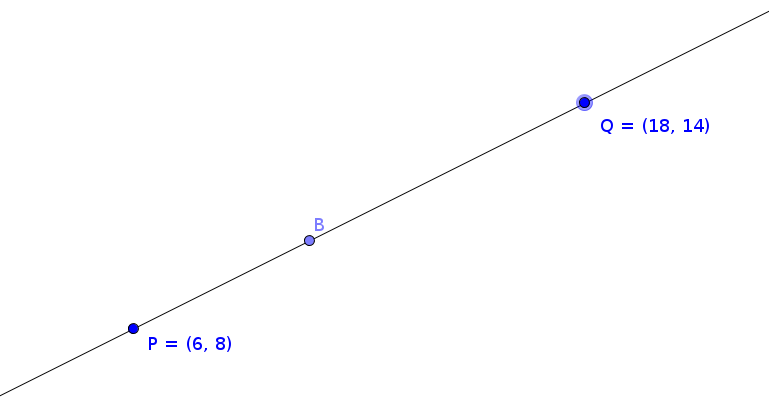Get Free Study Materials + 1 Week Free Trial of BrainsPrep Class 10 English Medium Tuition

Qn 14.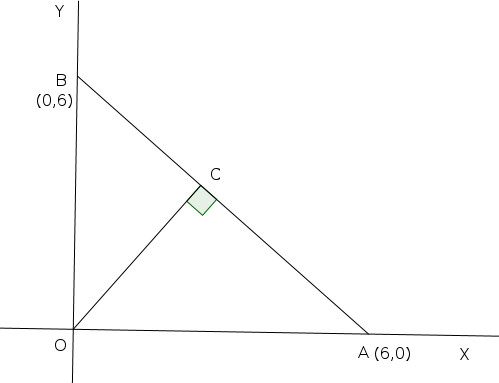In the figure, OC is perpendicular to AB.

(a) Prove that Δ OAB is isosceles ?

(b) Find the coordinates of C ?

(c) Write the equation of the line OC.

(a) OA = 6 ,OB =

Get Free Study Materials + 1 Week Free Trial of BrainsPrep Class 10 English Medium Tuition

Qn 15.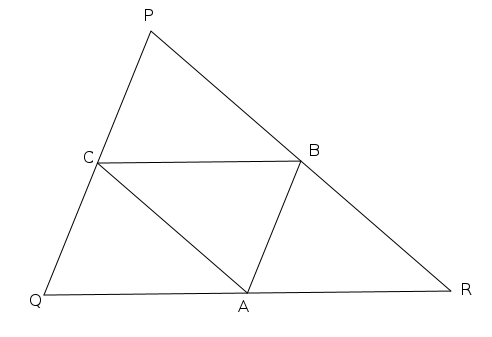In the figure A , B , C are the mid points of QR , PR , and PQ respectively.

A (2,4) R (5,5) B(4,7) .Then write the coordinates of P , Q , and C.

C (1, 6)

Q (-1,

Get Free Study Materials + 1 Week Free Trial of BrainsPrep Class 10 English Medium Tuition

Qn 16.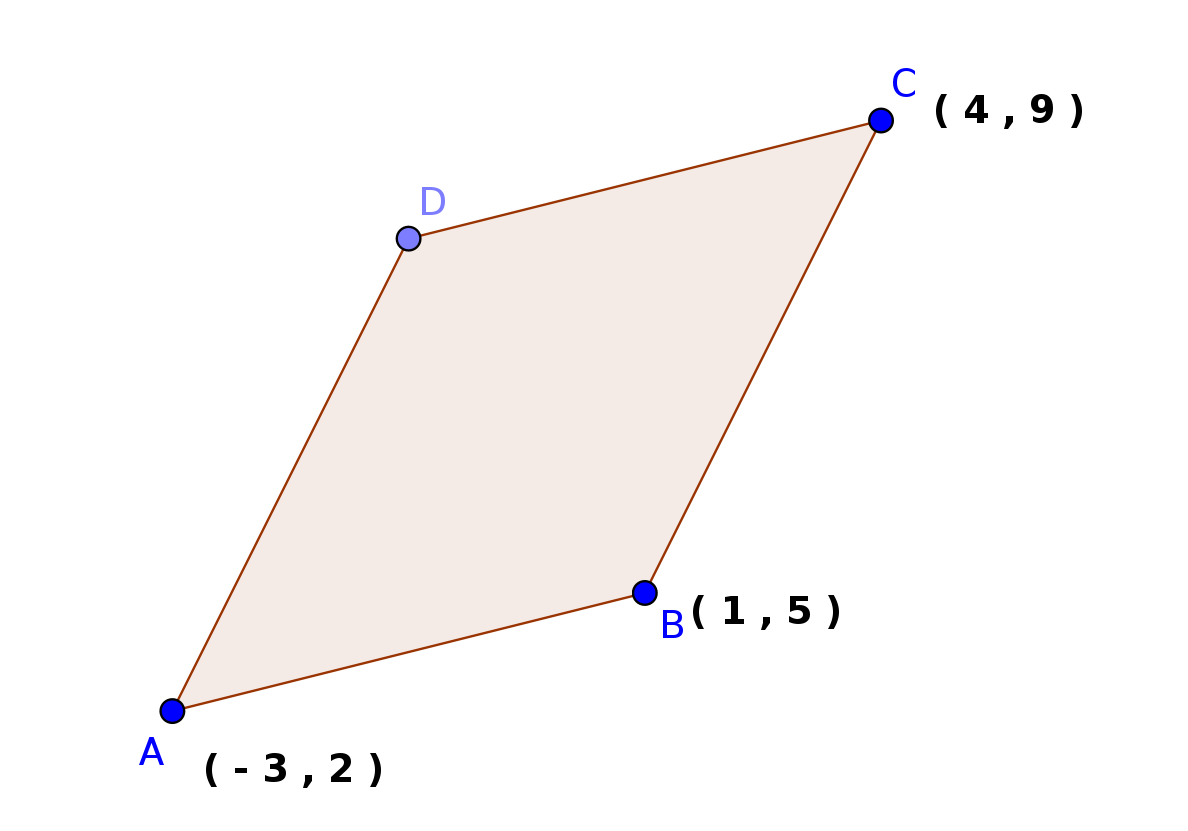The vertices of the parallelogram ABCD are A (-3 , 2) , B (1 , 5) ,C (4 , 9) Then

(a) Write the coordinates of D?

(b) Find the length of AB and AD

(c) Calculate the area of the parallelogram ?

Get Free Study Materials + 1 Week Free Trial of BrainsPrep Class 10 English Medium Tuition

Qn 17.

In the figure ABCD is a parallelogram. Write the coordinates of point C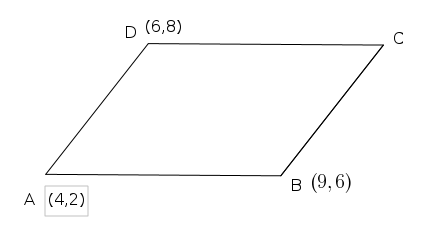X coordinate of point C = 9 + 6 - 4 = 11

Y coordinate of point C = 8 + 6 - 2 =

Get Free Study Materials + 1 Week Free Trial of BrainsPrep Class 10 English Medium Tuition